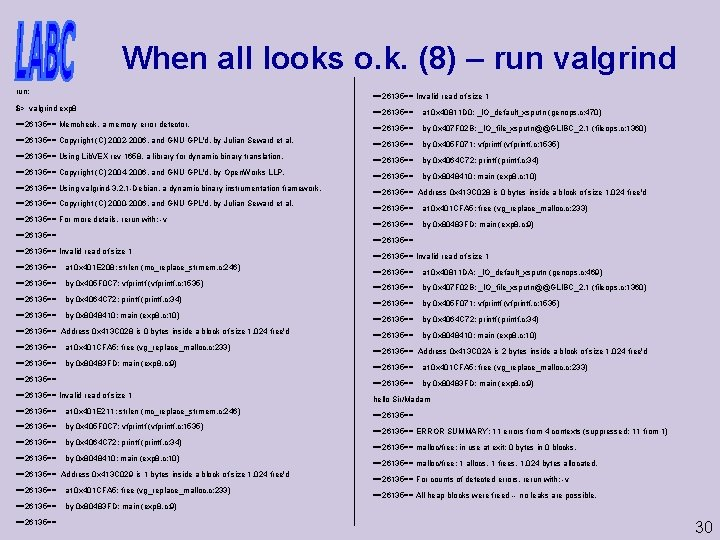# Valgrind tutorial Get install homepage http www valgrind

• Slides: 32Valgrind tutorialGet & install homepage: http: //www. valgrind. org/ install: extract: bzip 2 -d valgrind-XYZ. tar. bz 2 tar -xf valgrind-XYZ. tar which will create a directory called valgrind-XYZ; change into that directory and run: . /configure make install Many linux dists. come with prepared package, google 'dist-name valgrind' 2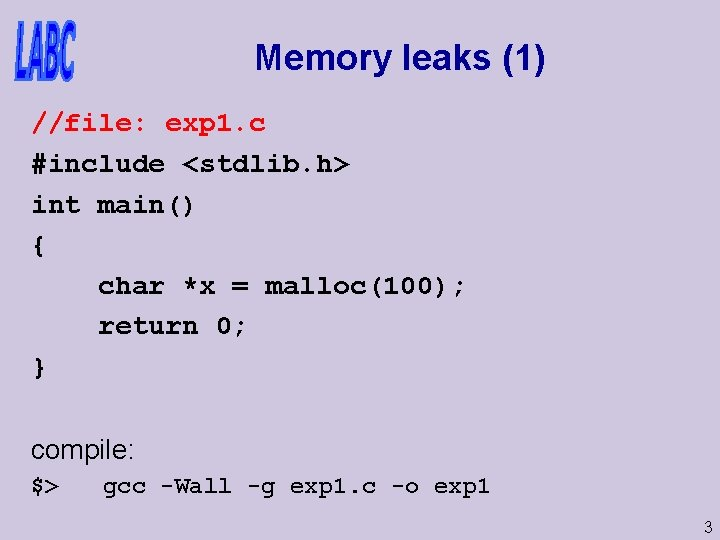Memory leaks (1) //file: exp 1. c #include <stdlib. h> int main() { char *x = malloc(100); return 0; } compile: \$> gcc -Wall -g exp 1. c -o exp 1 3Memory leaks (1) - code //file: exp 1. c #include <stdlib. h> int main() { char *x = malloc(100); return 0; } compile: \$> gcc -Wall -g exp 1. c -o exp 1 // -g for debug inf. 4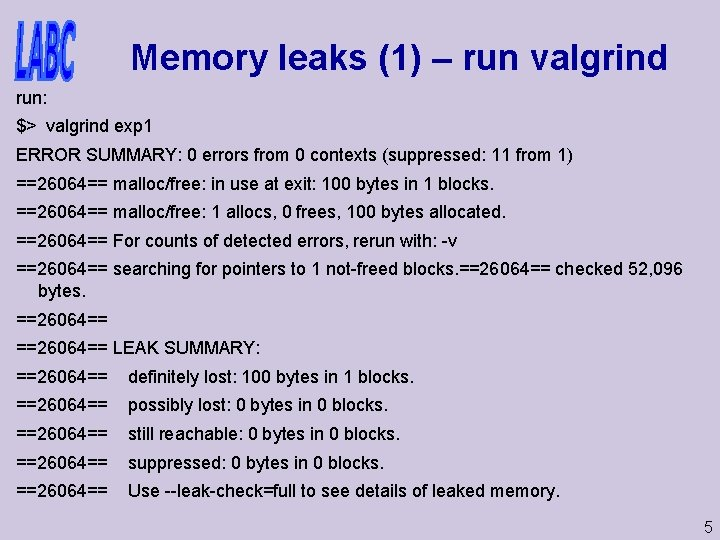Memory leaks (1) – run valgrind run: \$> valgrind exp 1 ERROR SUMMARY: 0 errors from 0 contexts (suppressed: 11 from 1) ==26064== malloc/free: in use at exit: 100 bytes in 1 blocks. ==26064== malloc/free: 1 allocs, 0 frees, 100 bytes allocated. ==26064== For counts of detected errors, rerun with: -v ==26064== searching for pointers to 1 not-freed blocks. ==26064== checked 52, 096 bytes. ==26064== LEAK SUMMARY: ==26064== definitely lost: 100 bytes in 1 blocks. ==26064== possibly lost: 0 bytes in 0 blocks. ==26064== still reachable: 0 bytes in 0 blocks. ==26064== suppressed: 0 bytes in 0 blocks. ==26064== Use --leak-check=full to see details of leaked memory. 5Memory leaks (1) – run valgrind run: \$> valgrind exp 1 ERROR SUMMARY: 0 errors from 0 contexts (suppressed: 11 from 1) ==26064== malloc/free: in use at exit: 100 bytes in 1 blocks. ==26064== malloc/free: 1 allocs, 0 frees, 100 bytes allocated. ==26064== For counts of detected errors, rerun with: -v ==26064== searching for pointers to 1 not-freed blocks. ==26064== checked 52, 096 bytes. ==26064== LEAK SUMMARY: ==26064== definitely lost: 100 bytes in 1 blocks. ==26064== possibly lost: 0 bytes in 0 blocks. ==26064== still reachable: 0 bytes in 0 blocks. ==26064== suppressed: 0 bytes in 0 blocks. ==26064== Use --leak-check=full to see details of leaked memory. 6Memory leaks (1) – run valgrind run: \$> valgrind exp 1 ERROR SUMMARY: 0 errors from 0 contexts (suppressed: 11 from 1) ==26064== malloc/free: in use at exit: 100 bytes in 1 blocks. ==26064== malloc/free: 1 allocs, 0 frees, 100 bytes allocated. ==26064== For counts of detected errors, rerun with: -v ==26064== searching for pointers to 1 not-freed blocks. ==26064== checked 52, 096 bytes. ==26064== LEAK SUMMARY: ==26064== definitely lost: 100 bytes in 1 blocks. ==26064== possibly lost: 0 bytes in 0 blocks. ==26064== still reachable: 0 bytes in 0 blocks. ==26064== suppressed: 0 bytes in 0 blocks. ==26064== Use --leak-check=full to see details of leaked memory. 7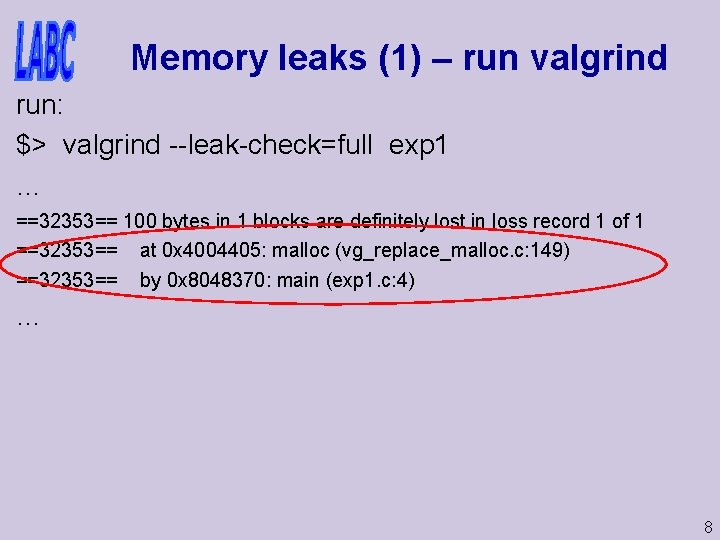Memory leaks (1) – run valgrind run: \$> valgrind --leak-check=full exp 1 … ==32353== 100 bytes in 1 blocks are definitely lost in loss record 1 of 1 ==32353== at 0 x 4004405: malloc (vg_replace_malloc. c: 149) ==32353== by 0 x 8048370: main (exp 1. c: 4) … 8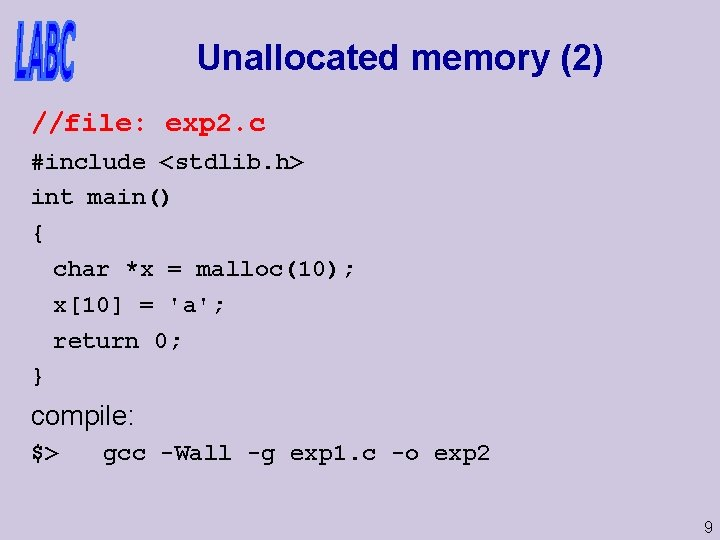Unallocated memory (2) //file: exp 2. c #include <stdlib. h> int main() { char *x = malloc(10); x = 'a'; return 0; } compile: \$> gcc -Wall -g exp 1. c -o exp 2 9Unallocated mem (2) – run valgrind run: \$> valgrind exp 2 ==26190== Invalid write of size 1 ==26190== at 0 x 804837 A: main (exp 2. c: 6) ==26190== Address 0 x 413 C 032 is 0 bytes after a block of size 10 alloc'd ==26190== at 0 x 401 D 38 B: malloc (vg_replace_malloc. c: 149) ==26190== by 0 x 8048370: main (exp 2. c: 5) ==26190== ERROR SUMMARY: 1 errors from 1 contexts (suppressed: 11 from 1) 10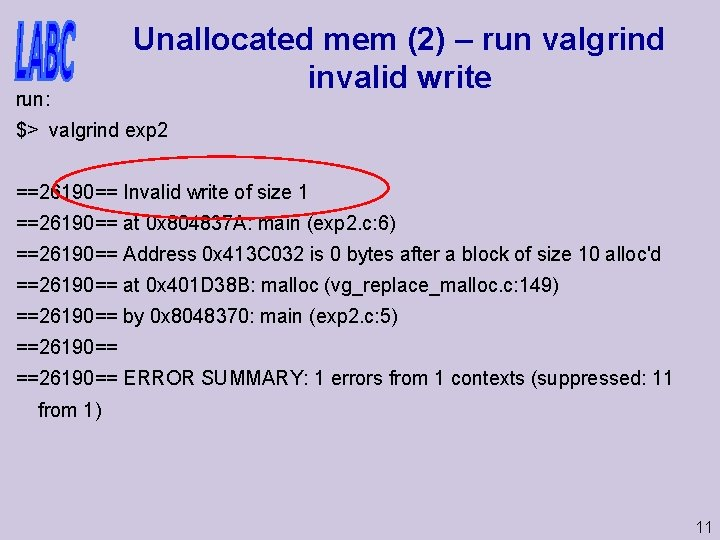run: Unallocated mem (2) – run valgrind invalid write \$> valgrind exp 2 ==26190== Invalid write of size 1 ==26190== at 0 x 804837 A: main (exp 2. c: 6) ==26190== Address 0 x 413 C 032 is 0 bytes after a block of size 10 alloc'd ==26190== at 0 x 401 D 38 B: malloc (vg_replace_malloc. c: 149) ==26190== by 0 x 8048370: main (exp 2. c: 5) ==26190== ERROR SUMMARY: 1 errors from 1 contexts (suppressed: 11 from 1) 11run: Unallocated mem (2) – run valgrind invalid write – where? \$> valgrind exp 2 ==26190== Invalid write of size 1 ==26190== at 0 x 804837 A: main (exp 2. c: 6) ==26190== Address 0 x 413 C 032 is 0 bytes after a block of size 10 alloc'd ==26190== at 0 x 401 D 38 B: malloc (vg_replace_malloc. c: 149) ==26190== by 0 x 8048370: main (exp 2. c: 5) ==26190== ERROR SUMMARY: 1 errors from 1 contexts (suppressed: 11 from 1) 12Uninitialized values (3) //file: exp 3. c #include <stdio. h> int main() { int x; if(x == 0) { printf("X is zero"); } return 0; } 13Uninitialized values (3) – run valgrind run: \$> valgrind exp 3 ==26127== Conditional jump or move depends on uninitialised value(s) ==26127== at 0 x 8048369: main (exp 3. c: 6) 14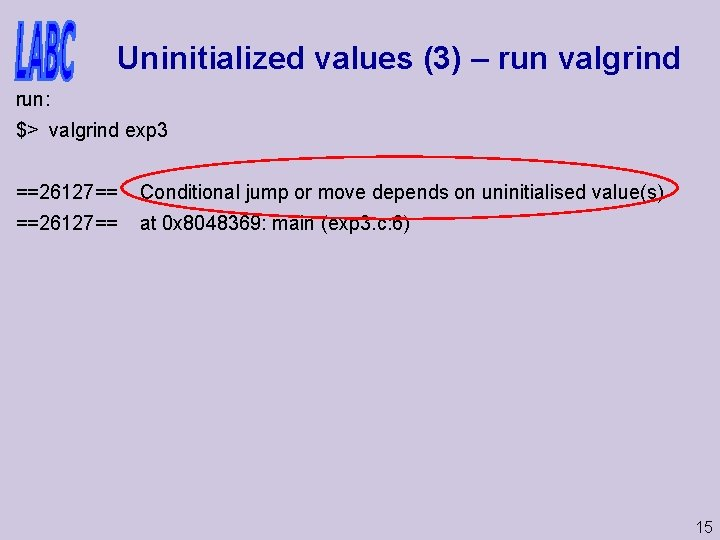Uninitialized values (3) – run valgrind run: \$> valgrind exp 3 ==26127== Conditional jump or move depends on uninitialised value(s) ==26127== at 0 x 8048369: main (exp 3. c: 6) 15run: Uninitialized values (3) – run valgrind where? \$> valgrind exp 3 ==26127== Conditional jump or move depends on uninitialised value(s) ==26127== at 0 x 8048369: main (exp 3. c: 6) 16Uninitialized values (4) //file: exp 4. c #include <stdio. h> int foo(int x) { if(x < 10) { printf("x is less than 10n"); } return 0; } int main() { int y; foo(y); return 0; } 17Uninitialized values (4) – run valgrind run: \$> valgrind exp 4 ==26128== Conditional jump or move depends on uninitialised value(s) ==26128== at 0 x 804835 E: foo (exp 4. c: 5) ==26128== by 0 x 804838 E: main (exp 4. c: 15) 18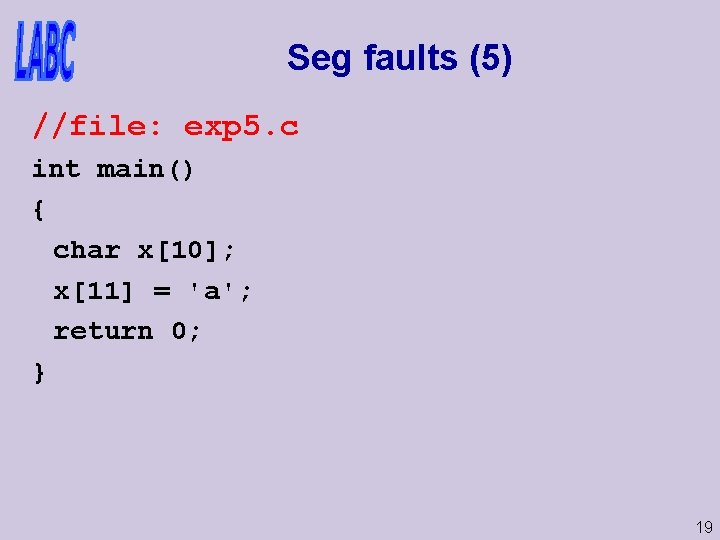Seg faults (5) //file: exp 5. c int main() { char x; x = 'a'; return 0; } 19Seg faults (5) – run valgrind run: \$> valgrind exp 5 ==26131== Invalid read of size 4 ==26131== at 0 x 8048346: main (exp 5. c: 6) ==26131== Address 0 x. BE 9561 BC is not stack'd, malloc'd or (recently) free'd 20Seg faults (5) – run valgrind run: \$> valgrind exp 5 ==26131== Process terminating with default action of signal 11 (SIGSEGV) ==26131== Access not within mapped region at address 0 x. BE 9561 BC ==26131== at 0 x 8048346: main (exp 5. c: 6) ==26131== Process terminating with default action of signal 11 (SIGSEGV) ==26131== Access not within mapped region at address 0 x. BE 9561 B 8 ==26131== at 0 x 40191 E 0: _vgn. U_freeres (vg_preloaded. c: 56) ==26131== ERROR SUMMARY: 1 errors from 1 contexts (suppressed: 11 from 1) 21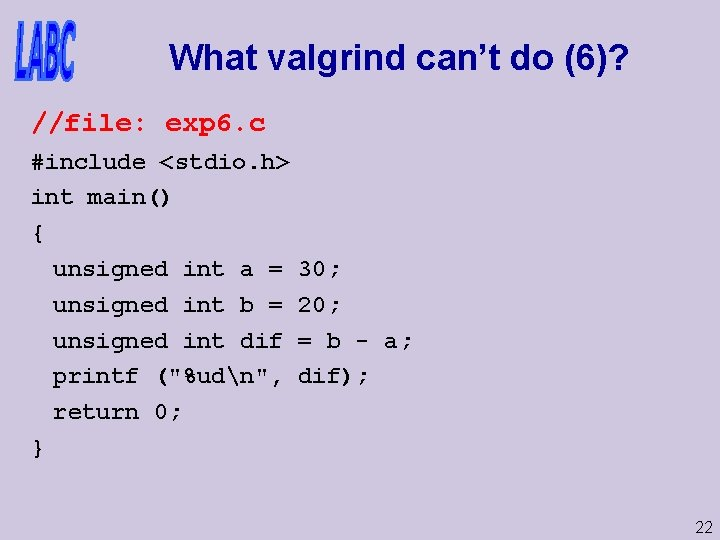What valgrind can’t do (6)? //file: exp 6. c #include <stdio. h> int main() { unsigned int a = unsigned int b = unsigned int dif printf ("%udn", return 0; } 30; 20; = b - a; dif); 22What valgrind can’t do (6)? run: \$> valgrind exp 6 ==26132== 4294967286 d ==26132== ERROR SUMMARY: 0 errors from 0 contexts (suppressed: 11 from 1) 23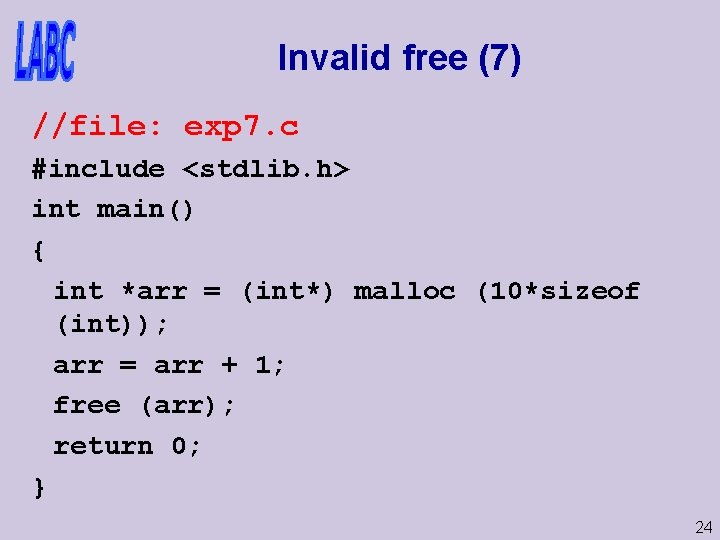Invalid free (7) //file: exp 7. c #include <stdlib. h> int main() { int *arr = (int*) malloc (10*sizeof (int)); arr = arr + 1; free (arr); return 0; } 24Invalid free (7) – run valgrind run: \$> valgrind exp 7 ==26133== Invalid free() / delete[] ==26133== at 0 x 401 CFA 5: free (vg_replace_malloc. c: 233) ==26133== by 0 x 80483 C 2: main (exp 7. c: 9) ==26133== Address 0 x 413 C 02 C is 4 bytes inside a block of size 40 alloc'd ==26133== at 0 x 401 D 38 B: malloc (vg_replace_malloc. c: 149) ==26133== by 0 x 80483 B 0: main (exp 7. c: 5) +=26133== ==26133== ERROR SUMMARY: 1 errors from 1 contexts (suppressed: 11 from 1) ==26133== malloc/free: in use at exit: 40 bytes in 1 blocks. ==26133== malloc/free: 1 allocs, 1 frees, 40 bytes allocated. 25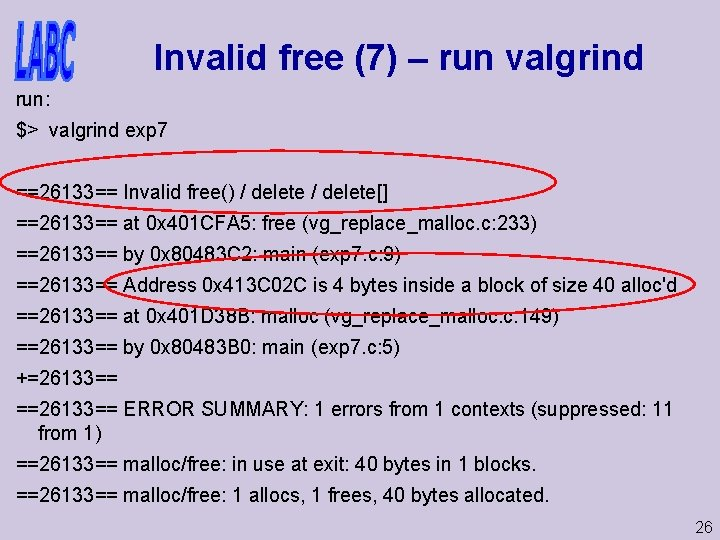Invalid free (7) – run valgrind run: \$> valgrind exp 7 ==26133== Invalid free() / delete[] ==26133== at 0 x 401 CFA 5: free (vg_replace_malloc. c: 233) ==26133== by 0 x 80483 C 2: main (exp 7. c: 9) ==26133== Address 0 x 413 C 02 C is 4 bytes inside a block of size 40 alloc'd ==26133== at 0 x 401 D 38 B: malloc (vg_replace_malloc. c: 149) ==26133== by 0 x 80483 B 0: main (exp 7. c: 5) +=26133== ==26133== ERROR SUMMARY: 1 errors from 1 contexts (suppressed: 11 from 1) ==26133== malloc/free: in use at exit: 40 bytes in 1 blocks. ==26133== malloc/free: 1 allocs, 1 frees, 40 bytes allocated. 26When all looks o. k. (8) //file: exp 8. c #include <stdio. h> #include <stdlib. h> #include <string. h> int main( int argc, char ** argv ) { char * sn. Greeting = malloc( sizeof(char) * 1024 ); strcpy( sn. Greeting, "hello" ); free(sn. Greeting); printf( "%s Sir/Madamn", sn. Greeting ); return 0; } 27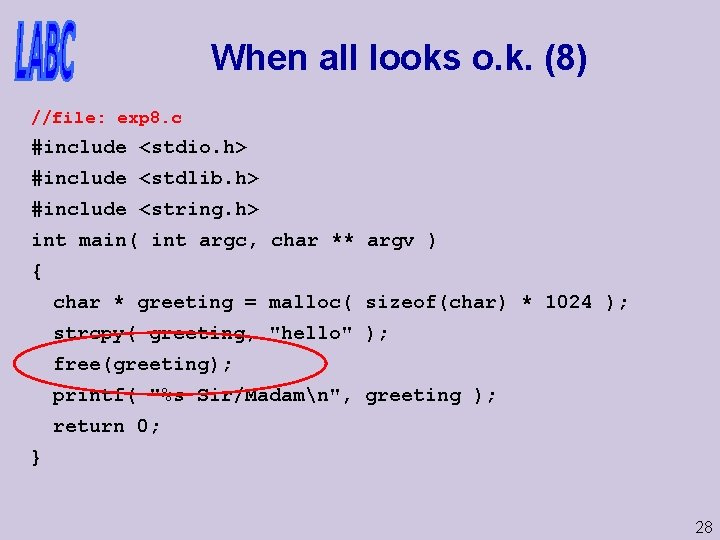When all looks o. k. (8) //file: exp 8. c #include <stdio. h> #include <stdlib. h> #include <string. h> int main( int argc, char ** argv ) { char * greeting = malloc( sizeof(char) * 1024 ); strcpy( greeting, "hello" ); free(greeting); printf( "%s Sir/Madamn", greeting ); return 0; } 28When all looks o. k. (8) – run: \$> exp 8 hello Sir/Madam \$> And with valgrind: 29